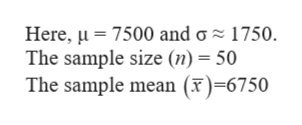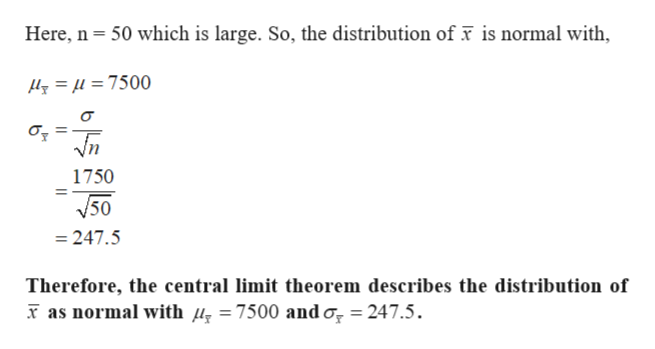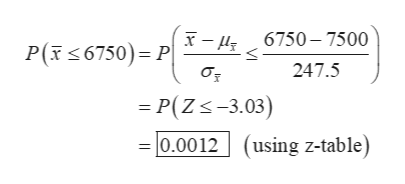Question

Workers at a large toxic cleanup project are concerned that their white blood cell counts may have been reduced. Let x be a random variable that represents white blood cell count per cubic millimeter of whole blood in a healthy adult. Then μ = 7500 and σ ≈ 1750.†

A random sample of n = 50 workers from the toxic cleanup site were given a blood test that showed x = 6750. What is the probability that, for healthy adults ,x will be this low or lower?

(a) How does the central limit theorem apply? Explain.
1The central limit theorem describes the distribution of x as normal with mean μ x = 7500 and σ x ≈1750.0.
The central limit theorem describes the distribution of x as normal with mean μ x = 7500 and σ x≈35.0.
The central limit theorem does not apply because the sample size is too small. The central limit theorem describes the distribution of x as normal with mean μ x = 7500 and σ x ≈247.5.

(b) Compute
P(x ≤ 6750).
(Round your answer to four decimal places.)
P(x ≤ 6750)
= 2

(c) Based on your answer to part (b), would you recommend that additional facts be obtained, or would you recommend that the workers' concerns be dismissed? Explain.
3
Yes, it would be reasonable to gather additional facts. The probability that the 50 workers' average white blood cell count is 6750 or lower, if they were healthy adults, is extremely low. Yes, it would be reasonable to gather additional facts. The probability that the 50 workers' average white blood cell count is 6750 or lower, if they were healthy adults, is extremely large.      No, it would not be necessary to gather additional facts. The probability that the 50 workers' average white blood cell count is 6750 or lower, if they were healthy adults, is extremely low. No, it would not be necessary to gather additional facts. The probability that the 50 workers' average white blood cell count is 6750 or lower, if they were healthy adults, is extremely large.
Step 1

Let the random variable X be the white blood cell count per cubic millimetre of whole blood in a healthy adult.help_outlineImage TranscriptioncloseHere, 7500 and g 1750 The sample size (n) = 50 The sample mean (x)=6750 fullscreen
Step 2

(a)

According to the central limit theorem, the distribution of sample means is normal, when the sample size is sufficiently large.help_outlineImage TranscriptioncloseHere, n 50 which is large. So, the distribution of x is normal with, y=u =7500 Vn 1750 V50 247.5 Therefore, the central limit theorem describes the distribution of as normal with /, =7500 and o, 247.5 fullscreen
Step 3

(b)

The required proba...help_outlineImage Transcriptionclose- 6750-7500 P(6750) P σ 247.5 = P(Zs-3.03) 0.0012 (using z-table) fullscreen

Want to see the full answer?

See Solution

Want to see this answer and more?

Our solutions are written by experts, many with advanced degrees, and available 24/7

See Solution
Tagged in

Other